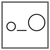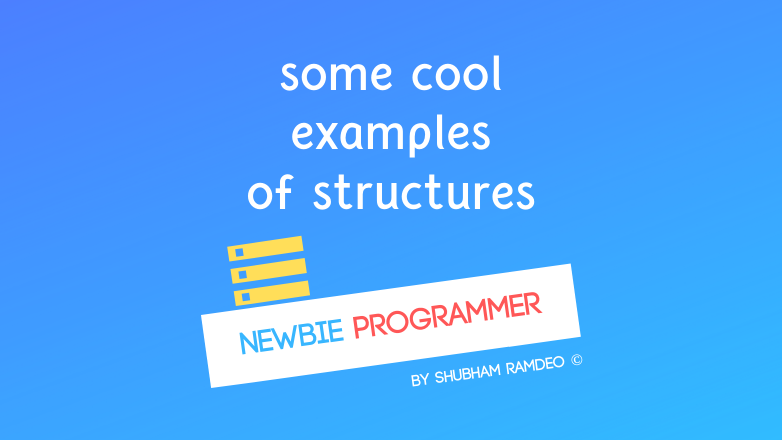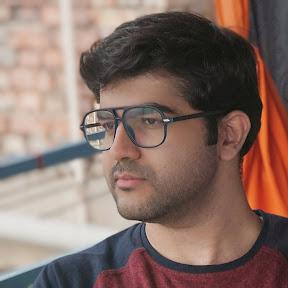Fuddlepixel coz tech is fun();

# Structure some Examples

Let us do some examples to illustrate the use of structure in as more real world like objects. Specially using nested structs and functions, macros too.

By Shubham Ramdeo
26 April 2016 · 3 mins read
# `c`  `newbie`That’s how we play with structures Practically !

Welcome to the Newbie Programmer Series. In the last part, we have covered everything important to know about structure. So I am quite confused what to discuss here ! Well let us do two examples explaining all the usage of structure so that you catch if you are having some trouble. So if you are new here, please check out all the previous parts (click here) to easily understand what is going down below.

### Finding distance between 2 points

[caption id=”” align=”aligncenter” width=”288”]The Distance Formula[/caption]

Let us make a simple program but with many old concepts revised. Say we have 2 points p1 and p2. To find the distance between two points, we use the formula :In our programming way, we can write :

``````d = sqrt( pow( (x2 - x1), 2) + pow( (y2 - y1), 2);
``````

NOTE : If you cannot get it, don’t worry. Do not concentrate and get scared by the formula. Do not think what that is. That’s the work of mathematicians. Our work is about  how to implement that in programming. Here we have used two functions, sqrt to find square root and pow to find power. Both of this are available in “math.h” library. So we will simply include them. Now check the complete code below.

``````#include "stdio.h" #include "math.h"
#define ADDPOINT(NAME, X, Y) point NAME; NAME.x=X; NAME.y=Y;

/*First, let us create a global definition of point
outside allbthe functions so that it can be accessed
by all the functions.*/
typedef struct {
int x;
int y;
} point;
/*our distance formula */
float distance( point p1, point p2 )
{
float d = sqrt( pow( (p2.x - p1.x), 2) + pow( (p2.y - p1.y), 2);
return d;
}
main ()
{
float dist = distance (A, B);
printf("The distance between points A and B is %f", dist);
printf("press enter to exit");
getchar();
}
``````

If you found it hard to understand what we have a done here with functions, please revise the functions and variables parts again so that you can understand how easy the above code is. I have simply include the required library files, then made the ADDPOINT macro to make the points easily. Then I described what a “point” is. Then I created a function called “distance”. It calculate the distance and return it. I have used floats values here so that we can get the exact values in decimals. And then the main function use all of them to print the answer. Simple.

### So many nested structures

Let us do an example to illustrate the use of multiple nested structs. Let us put a lot of real life objects, like GTA !

``````#include "stdio.h"
main()
{

/* These below are the interconnected structs.*/

typedef struct {
char name;
int birth;
} person;

typedef struct {
int price;
int pages;
person author;
} book;

typedef struct {
int year;
book textbooks;
person librarian;
} library;

/* Now using them */

//Making a Library
library schoolLibrary;

//Now giving it details
schoolLibrary.year = 2016;
schoolLibrary.librarian.name = "John the Great";
schoolLibrary.textbook.name = "Plane Trigonometry";
schoolLibrary.textbook.author.name = "S L Loney";

//using them, instead of writing long struct, assign a variable
int bdate = schoolLibrary.textbook.author.birth = 1860;
printf("%d", bdate);

//Not only limited to those big structs, make smaller as
person john;
john.birth = "1990";
}
``````

The above example is self-explanatory, I have used a lot of comments in them. I think that two are enough as we have discussed a lot of examples in the previous posts so need of more. And here the topic of structure ends. From the next post we will start something new. So stay connected.

##### Update me weekly ✉

Subscribe below and we’ll send you a weekly email summary of all new Code tutorials. Never miss out on learning about the next big thing.

#### Shubham RamdeoShubham Ramdeo is the founder of Fuddlepixel. He is a geek, a writer, involved with many technical and humanity projects.

Meet me!

#### Recent#### Related

`c`

`newbie`

Top